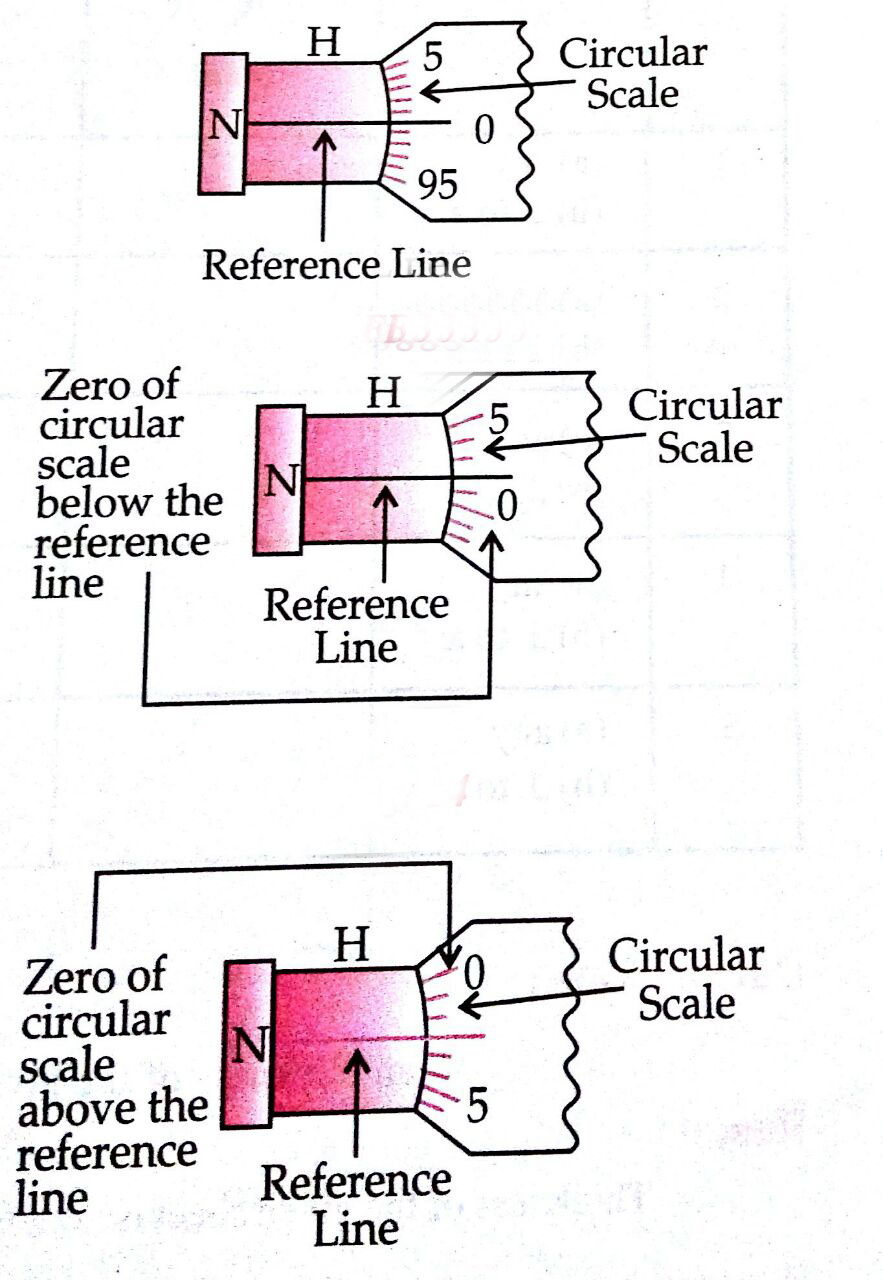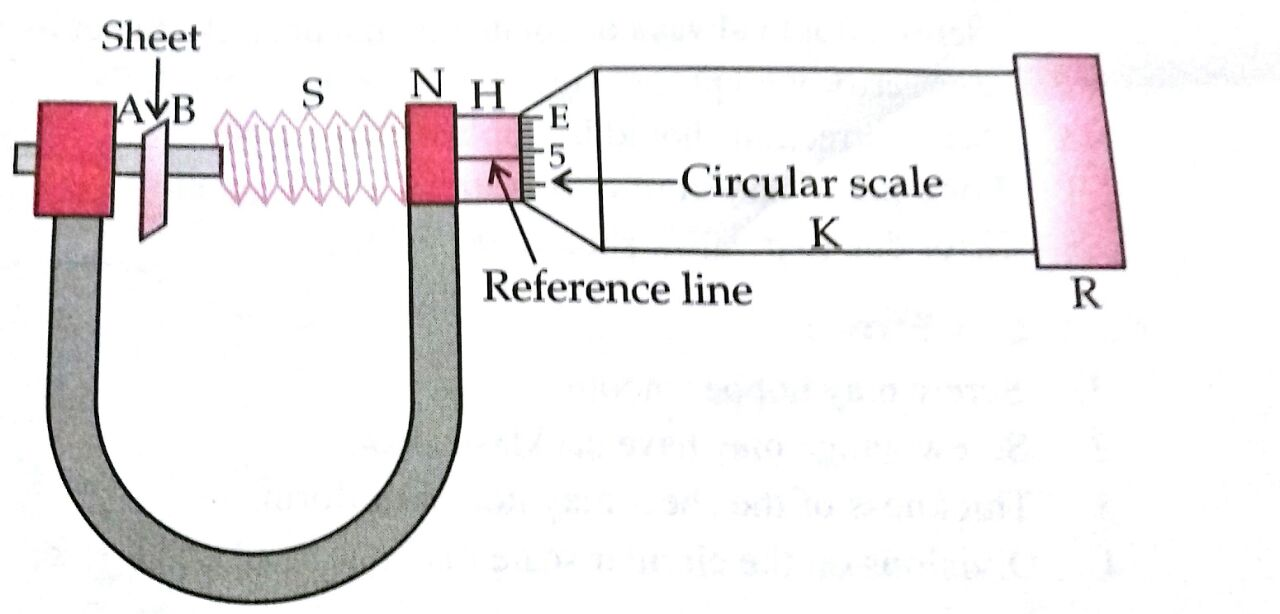# The least count of the main scale of a screw gauge is 1 mm. The minimum number of divisions on its circular scale required to measure 5  diameter of a wire is : Option 1) 50Option 2)200Option 3)100Option 4)500

C Chandbegamshaik

3

V Vinay Kumar

50

V Vishna Jwala

Ans.2, 200

To measure the thickness of the given sheet using screw gauge -1.    Note the number of divisions on the circular scale.

2.    Give five complete rotations to the screw.

3.    Note the linear distance moved by the screw.

4.    Find the pitch and L.C. of screw gauge.

5.    Find the zero error and zero correction by moving the screw only in one direction in such a way that studs A and B just touch each other.

6.    Now grip the given sheet in the gap A and B of the screw gauge.

7.    Turn the screw head till the ratchet arrangement gives a click.

8.    Note the readings of linear scale and circular scale and find the observed thickness using the relation, observation thickness = L.S.R. + C.S.R.

9.    Add the zero correction to the observed thickness to find the corrected diameter.

10.    Repeat steps 6 to 9 to find the thickness from four more different places.

- wherein

= M.S.R + C.S.R

L.C = least count

Least count =

&

So

Option 1)

50

Option 2)

200

Option 3)

100

Option 4)

500

Exams
Articles
Questions